.
.
.

## Age-specific fertility rates

Age-specific fertility rates for all birth orders combined and by birth order for the period 1994–2018 for Greenland are calculated on the basis of the official published live birth counts and population estimates (the de jure population).

Statistics Greenland combines 2 sources of information a) The Central Population Register(cpr.dk 1) and b) Birth certificates2 in cc to the National Board of Health in Nuuk

Everybody living in Greenland is assigned a 10-digit PIN(personal indentification number, danish: CPR) by birth or immigration. The system was introduced in Denmark in 1968, and from 1972 the same system was used in Greenland. All public administration uses the PIN.

For socioeconomic statistics this implys, that all administrative gathered information can be combined nationwide on all individuals with extremly high accuracy. Demographic measures can be calculated from microlevel using exact days.

The official aggregated tables on births are published in the StatBank of Statistics Greenland http://bank.stat.gl.

Full population account is also accessible in the statbank, at detailed Lexis-triangles level

Measures presented here are the most simple version of APC (Age, Period, Cohort) namely Age_Period as population exposures for a given age group are calculated as an average of population estimates for 1st January of a given year and the following year. As in this Lixes diagram:

if(!require(LexisPlotR)){install.packages("LexisPlotR")}
library(LexisPlotR)
lexis <- lexis_grid(year_start = 2015, year_end = 2020, age_start = 22, age_end = 25)
polygons <- data.frame(group = c(1, 1, 1, 1),
x = c("2017-01-01", "2017-01-01", "2018-01-01", "2018-01-01"),
y = c(23, 24, 24, 23))

lexis_polygon(lg = lexis, x = polygons$x, y = polygons$y, group = polygons$group)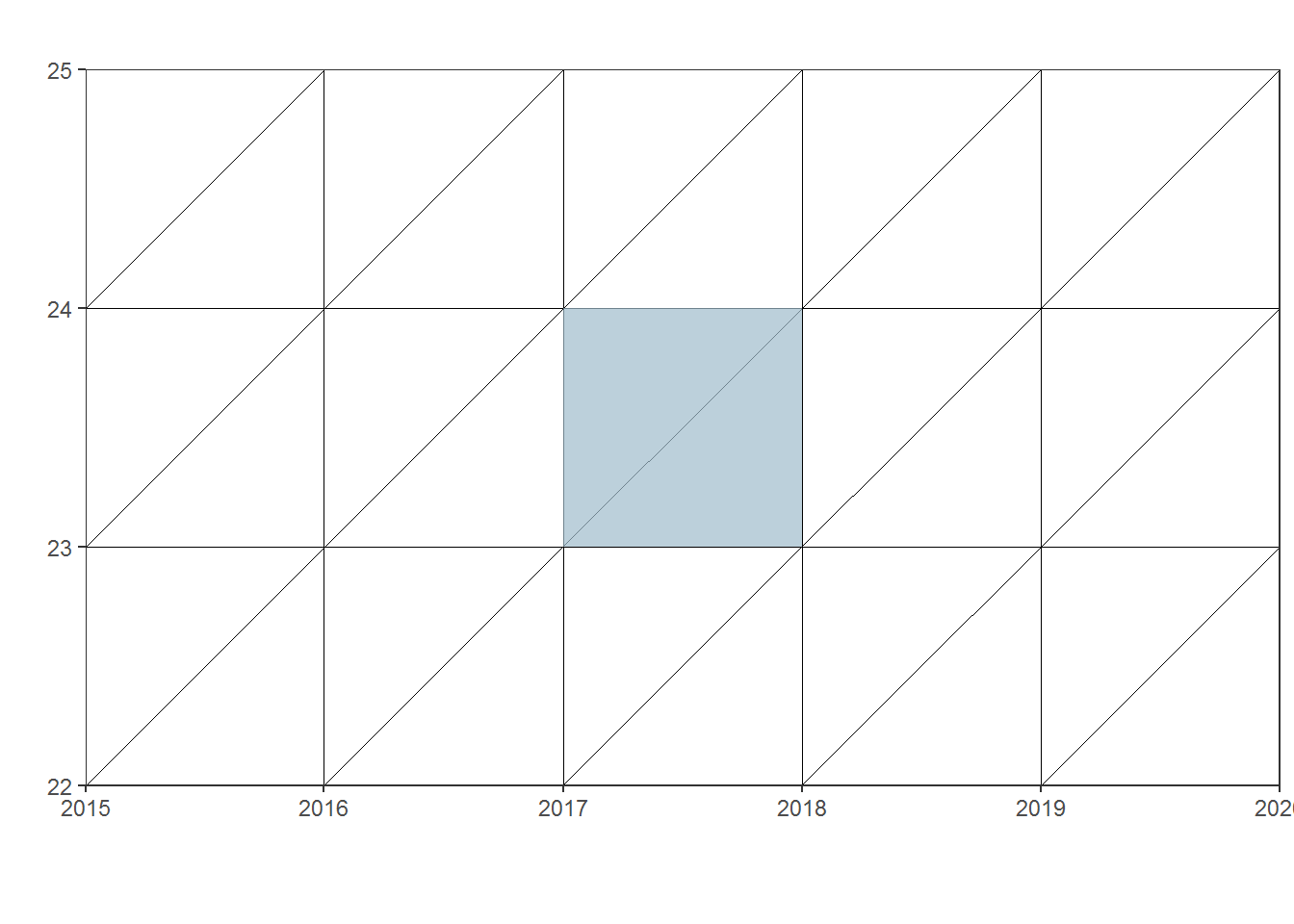## Births Live births, 1994–: Data downloaded on: 23 juni, 2020 On parity, the mothers ages are published from ≤14 to 49+, specified by one-year age groups. Birth order ranges from 1 through 8+. As can be seen in http://bank.stat.gl/BEEBBP if(!require(tidyverse)){install.packages("tidyverse")} library(tidyverse) if(!require(pxweb)){install.packages("pxweb")} library(pxweb) # PXWEB query & Download px_data <- pxweb_get(url = "http://bank.stat.gl/api/v1/en/Greenland/BE/BE10/BE1001/BE100120/BEXBBP.px", query = list("mothers place of birth"=c("*"), "mothers age"=c("*"), "parity"=c("*"), "time"=c("*")) ) # Convert to data.frame BIRTHS_pxw <- as.data.frame(px_data, column.name.type = "text", variable.value.type = "code")%>% as_tibble() BIRTHS = BIRTHS_pxw %>% spread('time','Livebirth') rm(px_data, BIRTHS_pxw) ## Female Population Female population, 1994–: Data downloaded on: 23 juni, 2020 Annual population estimates are publish early February every year and population exposures for a given age group are calculated as an average of population estimates for 1st January of a given year and the following year. Table can be found on http://bank.stat.gl/BEEST1 Alternatively it is also possible to find tables on mid-year population in the Statbank. Mid-year population is higher than January 1.st if(!require(tidyverse)){install.packages("tidyverse")} library(tidyverse) if(!require(pxweb)){install.packages("pxweb")} library(pxweb) # PXWEB query & Download px_data <- pxweb_get(url = "http://bank.stat.gl/api/v1/en/Greenland/BE/BE01/BE0120/BEXST1.PX", query = list("place of birth"=c("N","S"), "gender"=c("K"), "age"=c("*"), "time"=c("*")) ) # Convert to data.frame POP_F_pxw <- as.data.frame(px_data, column.name.type = "text", variable.value.type = "code")%>% as_tibble() # POP_F = POP_F_pxw %>% spread('time','Population January 1st') rm(px_data, POP_F_pxw) ## Calculations Age-specific fertility rates calculated on on-year age groups for a small population, as Greenland, will show large fluctuations from one year to the next. For each age, x, 14 - 49 a rate is calculated as: $f\left(x\right)=B\left(x{\right)}_{T}/\left(P\left(x\right){}_{T}+P\left(x\right){}_{T}{+}_{1}\right)/2$ where B is Livebirth and P i Population estimate. In 2017 the age-specific fertilityrate for greenlandic born women was: 49/398.5 = 0.122961 if(!require(LexisPlotR)){install.packages("LexisPlotR")} library(LexisPlotR) lexis2 <- lexis_grid(year_start = 2015, year_end = 2020, age_start = 22, age_end = 25) # Create a data.frame that contains the labels labels <- data.frame( x = c( as.Date("2016-11-01"), as.Date("2017-05-15"), as.Date("2017-05-15"), as.Date("2017-07-01"), as.Date("2018-03-01") ), y = c( 23.5, 23.7, 23.4, 23.2, 23.5 ), label = c( "395", "birth: 49", "exposure:", "398.5=(395+402)/2", "402" ) ) # Use geom_text to plot the labels lexis2 + geom_text(aes(x = labels$x, y = labels$y, label = labels$label))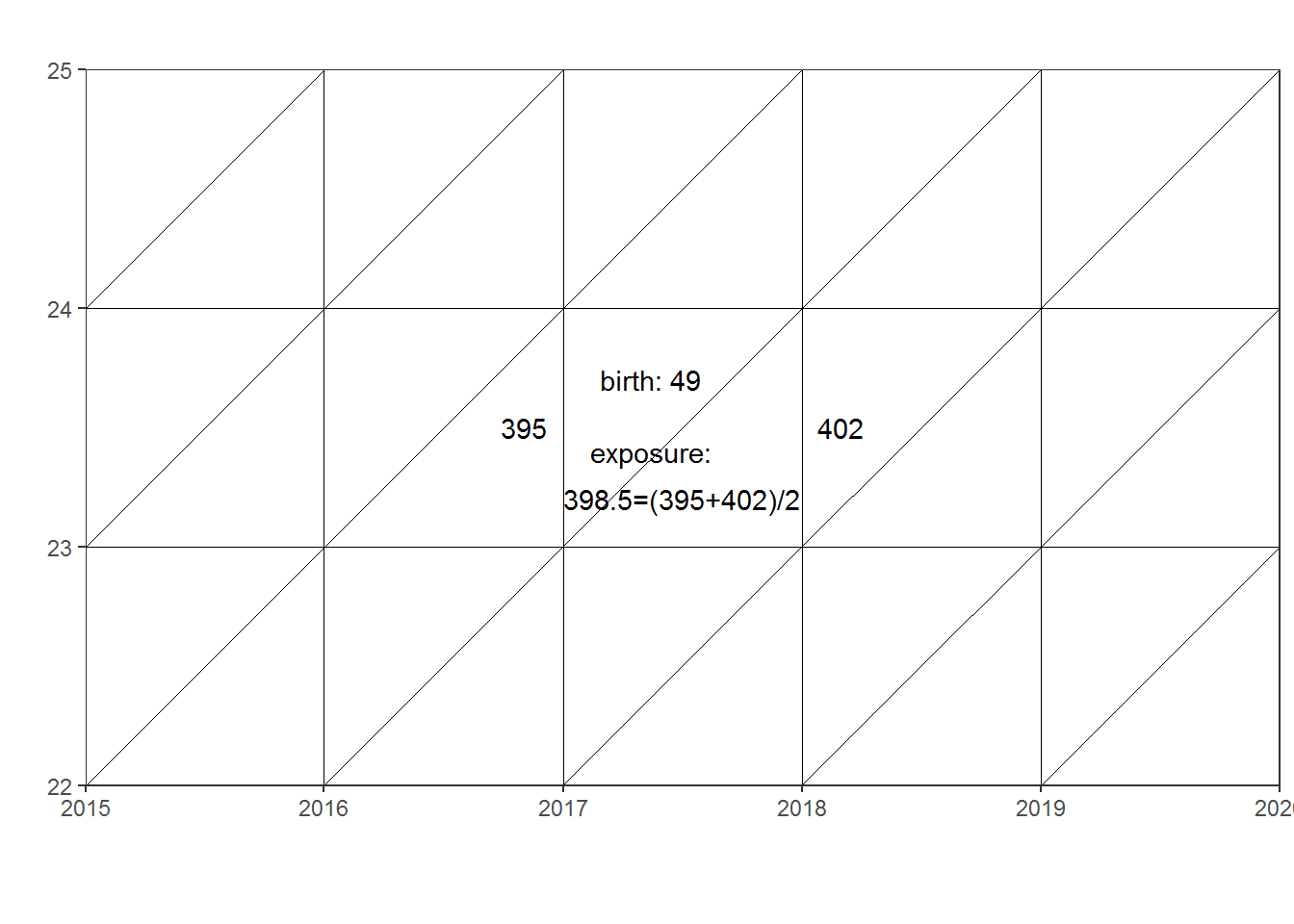Age-specific fertility rates calculated on one-year age groups for a small population, as Greenland, will show large fluctuations from one year to the next.

Here age 23 is plottet from 1994 and onward:

BIRTHS1 <- BIRTHS
BIRTHS1 <- BIRTHS1 %>% filter(BIRTHS1$mothers place of birth == "N") BIRTHS1 <- subset(BIRTHS1, select = -mothers place of birth) BIRTHS1$parity <- as.numeric(as.character(BIRTHS1$parity)) BIRTHS1$parity[BIRTHS1$parity>1]<-1 BIRTHS2<-subset(aggregate(BIRTHS1[,3:27], list(age=BIRTHS1$mothers age,BIRTHS1$parity),sum), select=-Group.2) rm(BIRTHS1) POP_F <- POP_F %>% filter(POP_F$place of birth == "N")
POP_F <- select(POP_F, age,1994,1995,1996,1997,1998,1999,
2000,2001,2002,2003,2004,2005,2006,2007,2008,2009,2010,
2010,2011,2012,2013,2014,2015,2016,2017,2018,2019)
POP_F$age <- as.numeric(as.character(POP_F$age))
POP_F <- filter(POP_F, age > 13, age < 50)

EXP<-POP_F[,1:27]
EXP[,2:26]<-(POP_F[,2:26]+POP_F[,3:27])/2
EXP$age<-as.numeric(as.character(EXP$age))

EXP<-POP_F[,1:ncol(POP_F)]
d1 <- EXP[,2:ncol(POP_F)-1]
d2 <-POP_F[,3:ncol(POP_F)-1]
d3 <- POP_F[,3:ncol(POP_F)]

EXP[,3:ncol(POP_F)-1]<-(POP_F[,3:ncol(POP_F)-1]+POP_F[,3:ncol(POP_F)])/2
EXP$age<-as.numeric(as.character(EXP$age))

ASFR2<-BIRTHS2
ASFR2[,2:26]<-ASFR2[,2:26]/EXP[,2:26]
ASFR2$age<-as.numeric(as.character(ASFR2$age))

TFR2<-colSums(ASFR2[,2:26])
ASFR3<-ASFR2
ASFR3[,2:26]<-ASFR3[,2:26]*ASFR3[,1]
MAB2<-colSums(ASFR3[,2:26])/TFR2+0.5

ASFR223 <- filter(ASFR2, age == '23')
ASFR23<-colSums(ASFR223[,2:26])

plot(ASFR23, type="l", xlab="time", ylab="rate")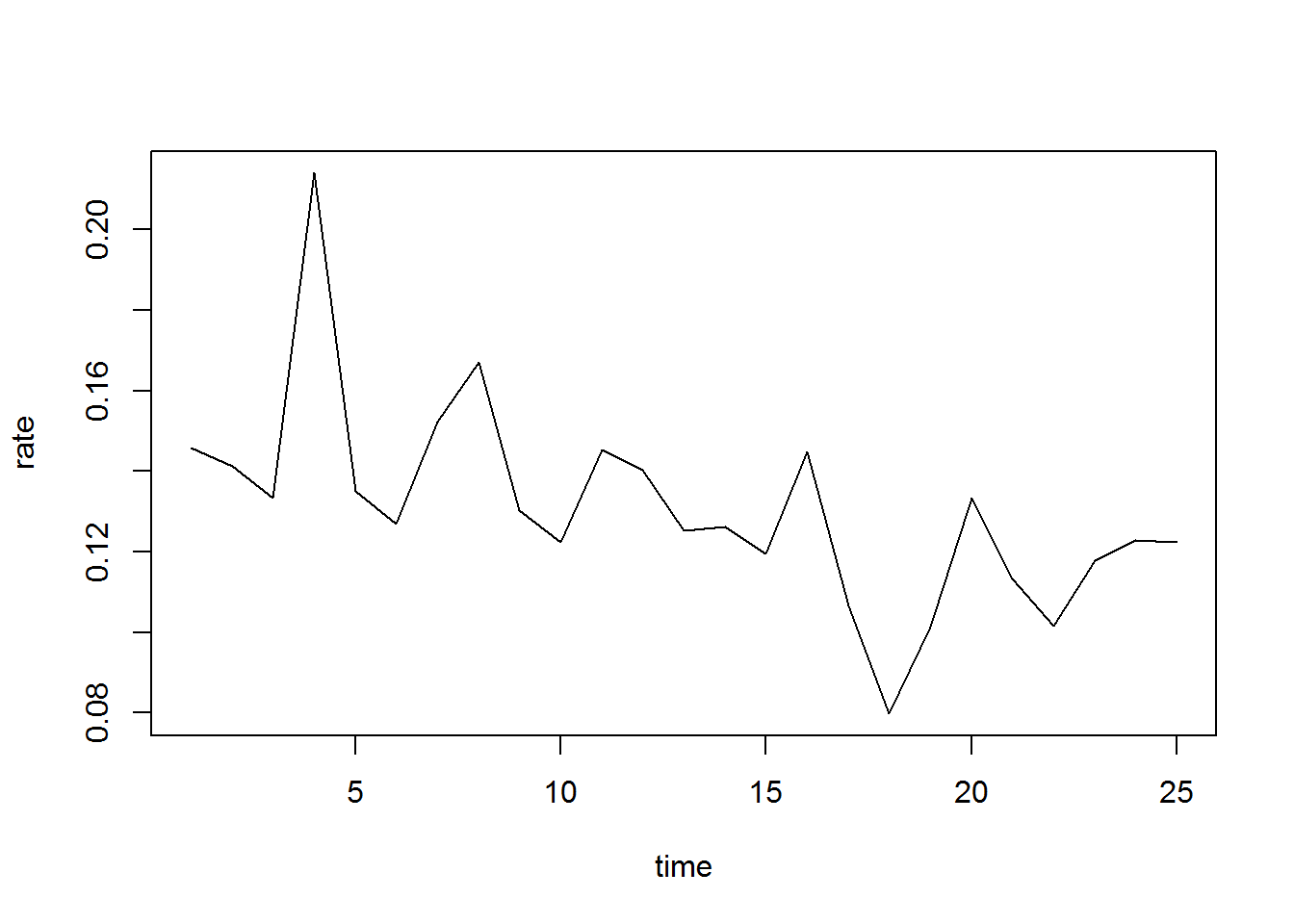ylab('rate')
## $y ##  "rate" ## ## attr(,"class") ##  "labels" ASFR2%>% gather(time,value,-age)%>% filter(time=='2018')%>% ggplot(aes(x=age,y=value))+ geom_line()+ geom_smooth() + labs(x = "age", y = "rate", title = "Age-specific fertility rates, 2018", subtitle = "annual flucturations due to a very small population", caption = "Statistics Greenland") + theme_statgl()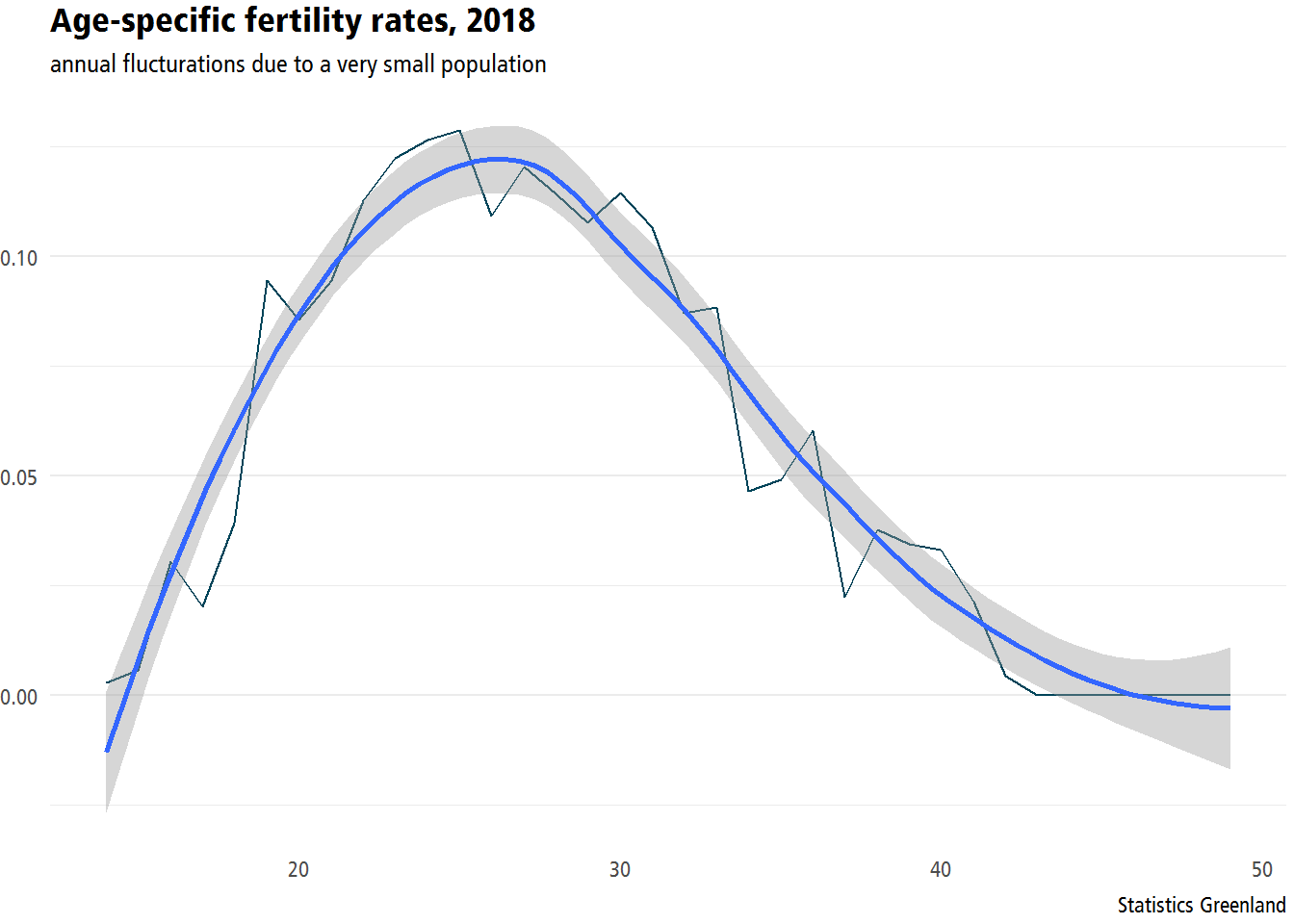Often, when dealing with small areas fertility rates are calculated for more than one year, for instance 5-year average, in a Lexis diagram: if(!require(LexisPlotR)){install.packages("LexisPlotR")} library(LexisPlotR) lexis3 <- lexis_grid(year_start = 2014, year_end = 2020, age_start = 22, age_end = 25) polygons3 <- data.frame(group = c(1, 1, 1, 1), x = c("2014-01-01", "2014-01-01", "2019-01-01", "2019-01-01"), y = c(23, 24, 24, 23)) lexis_polygon(lg = lexis3, x = polygons3$x, y = polygons3$y, group = polygons3$group)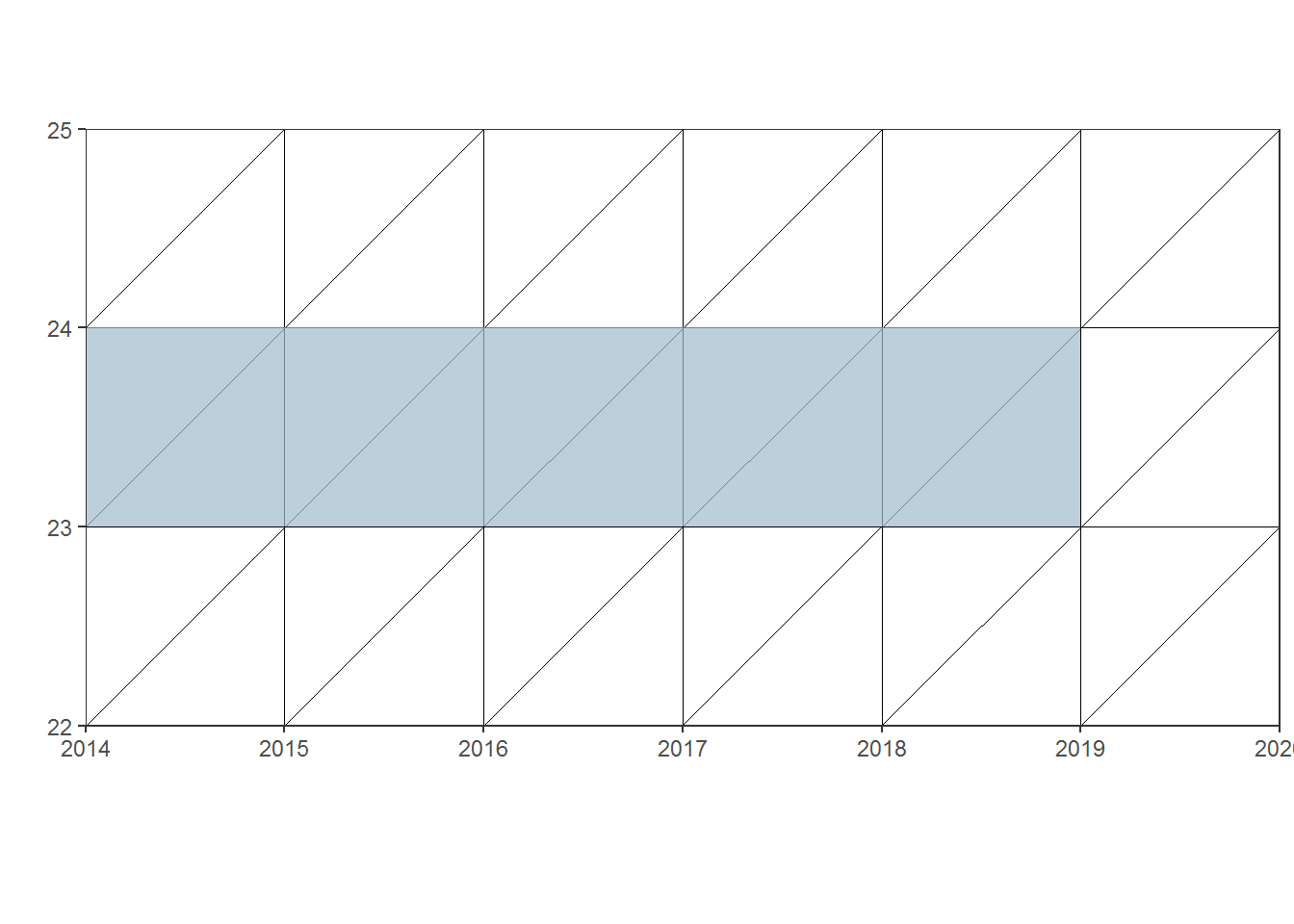if(!require(LexisPlotR)){install.packages("LexisPlotR")}
library(LexisPlotR)

# Create a data.frame that contains the labels
labels5 <- data.frame(
x = c(
as.Date("2014-03-01"),
as.Date("2015-03-01"),
as.Date("2016-03-01"),
as.Date("2017-03-01"),
as.Date("2018-03-01"),
as.Date("2019-03-01"),
as.Date("2014-05-15"),
as.Date("2015-05-15"),
as.Date("2016-05-15"),
as.Date("2017-05-15"),
as.Date("2018-05-15"),
as.Date("2016-05-15"),
as.Date("2016-05-15"),
as.Date("2016-07-01")
),
y = c(
23.5,
23.5,
23.5,
23.5,
23.5,
23.5,
23.7,
23.7,
23.7,
23.7,
23.7,
24.3,
22.8,
22.6
),
label = c(
"438",
"411",
"436",
"395",
"402",
"399",
"birth: 48",
"birth: 43",
"birth: 49",
"birth: 49",
"birth: 49",
"livebirth 2014-18: 238=48+43+49+49+49",
"exposure 2014-18:",
"2062.5=(438/2)+411+436+395+402+(399/2)"
)
)

lexis5 <- lexis_grid(year_start = 2014, year_end = 2020, age_start = 22, age_end = 25)

# Use geom_text to plot the labels
lexis5 + geom_text(aes(x = labels5$x, y = labels5$y, label = labels5$label))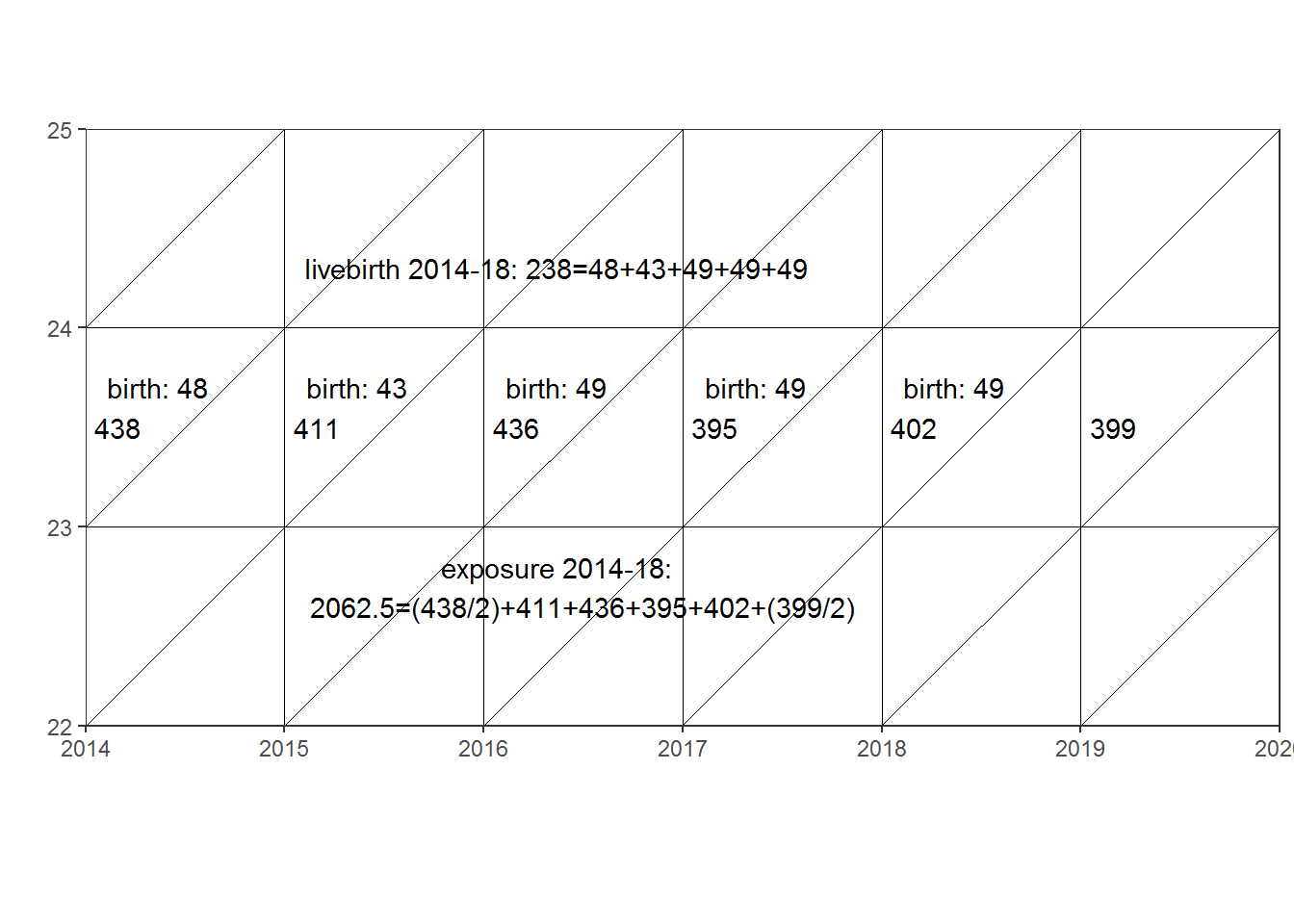In this example an agespecific fertility rate for women aged 23 can be calculated as 0,11539 = 238/2062.5 #5 year fertility #Birth 5 years a-groups Ba<-BIRTHS2[,1:26] #Ba <- Ba[,-c(2)] Ba[,2:22]<-(Ba[,2:22]+Ba[,3:23]+Ba[,4:24]+Ba[,5:25]+Ba[,6:26]) Ba$age<-as.numeric(as.character(Ba$age)) #Ba <- Ba[,-c(23:26)] #Exposure 5 years a-groups EXPa<-POP_F[,1:27] EXPa[,2:23]<-(POP_F[,2:23]/2+POP_F[,3:24]+POP_F[,4:25]+POP_F[,5:26]+POP_F[,6:27]/2) EXPa$age<-as.numeric(as.character(EXPa$age)) #EXPa <- EXPa[,-c(23:27)] ASFR2a<-Ba ASFR2a[,2:22]<-Ba[,2:22]/EXPa[,2:22] ASFR2a$age<-as.numeric(as.character(ASFR2a$age)) TFR2a<-colSums(ASFR2a[,2:26]) ASFR3a<-ASFR2a ASFR3a[,2:26]<-ASFR3a[,2:26]*ASFR3a[,1] MAB2a<-colSums(ASFR3a[,2:26])/TFR2a+0.5 ASFR223a <- filter(ASFR2a, age == '23') ASFR23a<-colSums(ASFR223a[,2:22]) plot(ASFR23a, type="l", xlab="time", ylab="rate")## Total fertility rate plot(TFR2, type="l", xlab="time", ylab="Total Fertility Rate ")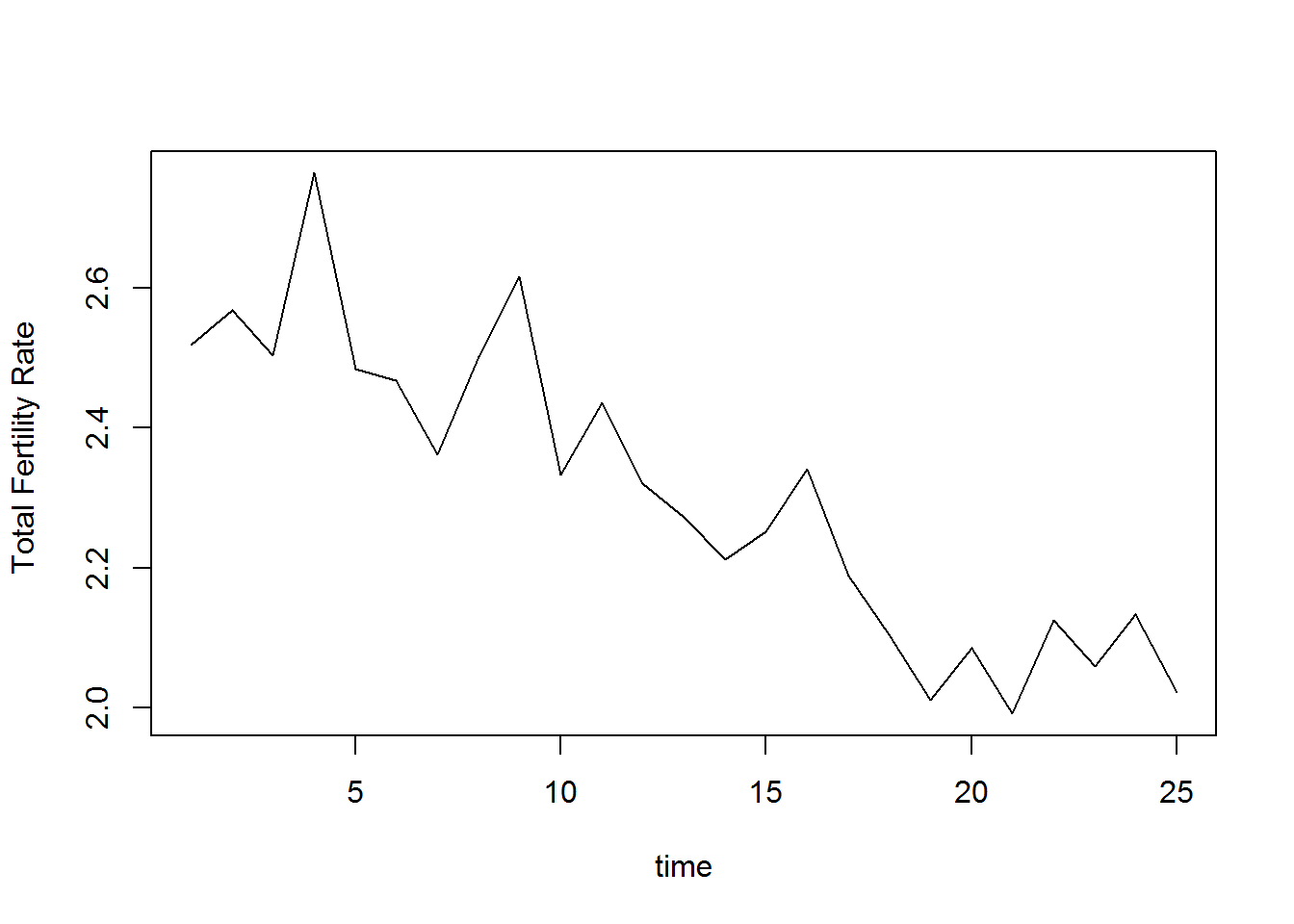EXP2<-EXP[EXP$age>=14&EXP$age<=49,c(1,2:26)] EXP_BO<-rbind(EXP2,EXP2,EXP2,EXP2,EXP2) BIRTHS1 <- BIRTHS BIRTHS1 <- BIRTHS1 %>% filter(BIRTHS1$mothers place of birth == "N")
BIRTHS1 <- subset(BIRTHS1, select = -mothers place of birth)
BIRTHS1$parity <- as.numeric(as.character(BIRTHS1$parity))
BIRTHS1$parity[BIRTHS1$parity>5]<-5
BIRTHS2<-subset(aggregate(BIRTHS1[,3:27],
list(as.numeric(as.character(BIRTHS1$mothers age)),BIRTHS1$parity),sum))

ASFR_BO<-BIRTHS2
ASFR_BO[,3:27]<-ASFR_BO[,3:27]/EXP_BO[,2:26]
TFR_BO<-aggregate(ASFR_BO[,3:27],
list(ASFR_BO$Group.2),sum) ASFR_BO2<-ASFR_BO ASFR_BO2[,3:27]<-ASFR_BO2[,3:27]*ASFR_BO[,1] MAB_BO<-aggregate(ASFR_BO2[,3:27], list(ASFR_BO2$Group.2),sum)
MAB_BO[,2:26]<-MAB_BO[,2:26]/TFR_BO[,2:26]+0.5

YLIM<-range(ASFR2[1:36,2:26])
YLIM2<-range(0,3)  #range(TFR1[1,2:43])
YLIM3<-range(0,700)  #range(TFR1[1,2:43])
AGES<-ASFR2[,1]
YEARS<-1977:2018
YEARS2<-1994:2018
YEARS3<-1973:2018

YEARSLast<-2018

for(Y in YEARSLast){
plot(AGES,ASFR2[1:36,Y-1994+2],type="l",col=1,lwd=2,lty=2,
xlab="Age",ylab="ASFR",main=Y,
ylim=YLIM,xaxs="i",yaxs="i",
panel.first=grid(col=1))
if(Y>=YEARS2){
lines(AGES,ASFR2[1:36,Y-1994+2],type="l",col=1,lwd=1.5,lty=1)
for(BO in 1:5){
lines(AGES,ASFR_BO[ASFR_BO$Group.2==BO,Y-1994+3],type="l",col=BO+1,lwd=1,lty=1) } } legend("topright",paste("ASFR",c("HFC","TOT",1:4,"5p"),sep="_"),col=c(1,1:6),lwd=c(2,1.5,1,1,1,1,1),lty=c(2,1,1,1,1,1,1)) }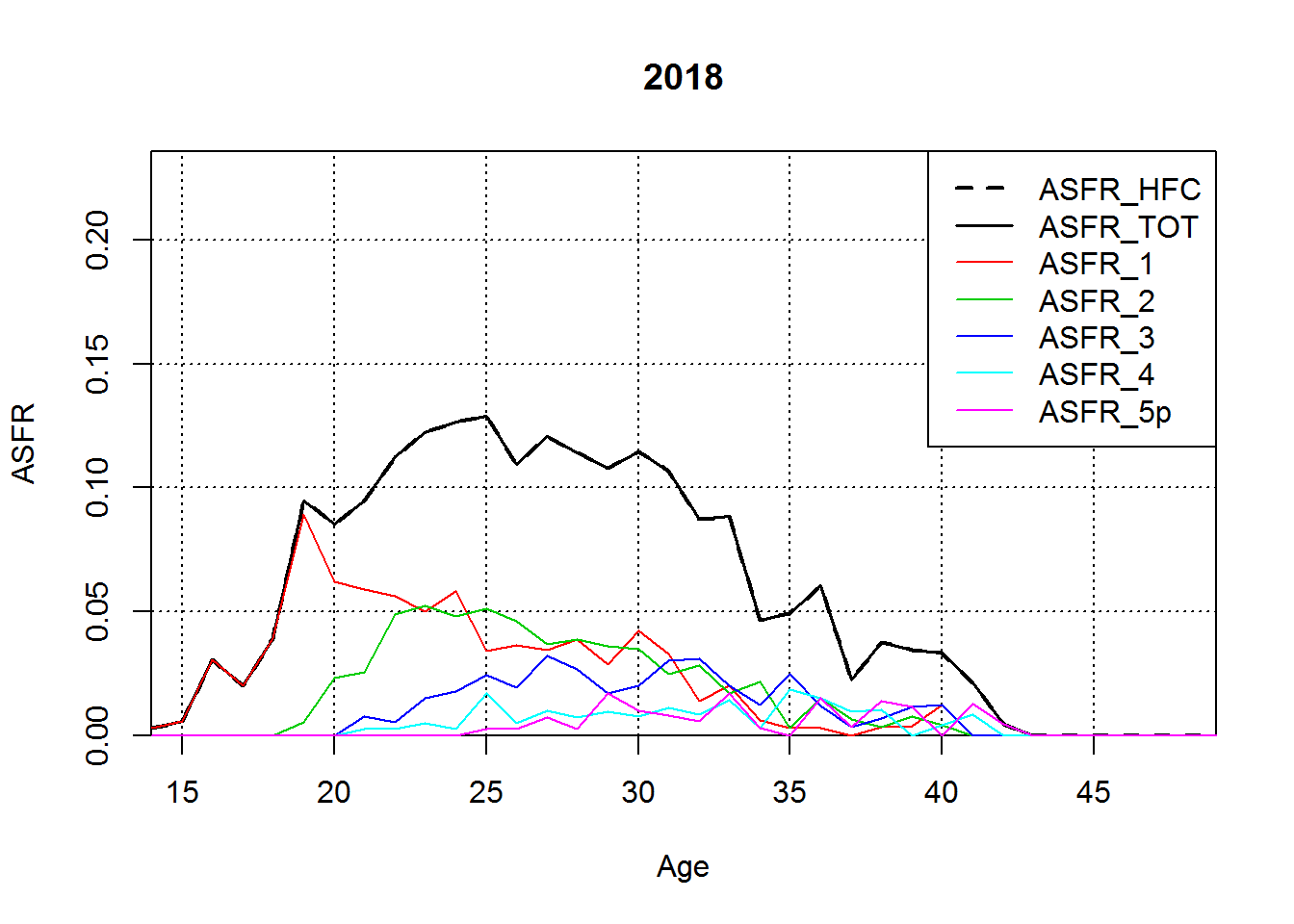plot(MAB_BO$Group.1,MAB_BO[1,2:26],type="l",col=1,lwd=2,lty=2, xlab="Year",ylab="MABi",main=YEARSLast, ylim=c(22,34),xaxs="i",yaxs="i", panel.first=grid(col=1)) for(BO in 1:4){ lines(MAB_BO$Group.1,MAB_BO[BO,2:26],type=“l”,col=BO+1,lwd=1,lty=1) } legend(“topright”,paste(“ASFR”,c(“HFC”,“TOT”,1:4,“5p”),sep="_"),col=c(1,1:6),lwd=c(2,1.5,1,1,1,1,1),lty=c(2,1,1,1,1,1,1)) ## Mothers mean age at birth plot(MAB2)#### HFC OUTPUT OUTPUTFILENAME<-“GRL_07_BO.csv” Country<-“GRL” Region<-NA Urban<-NA Origin<-NA Year1<-rep(YEARS2,each=length(AGES)) Year2<-Year1 Age<-AGES AgeInt<-1 AgeDef<-“ACY” Vitality<-1 ASFR1<-as.vector(unlist(ASFR_BO[ASFR_BO$Group.2==1,3:27])) ASFR2<-as.vector(unlist(ASFR_BO[ASFR_BO$Group.2==2,3:27])) ASFR3<-as.vector(unlist(ASFR_BO[ASFR_BO$Group.2==3,3:27])) ASFR4<-as.vector(unlist(ASFR_BO[ASFR_BO$Group.2==4,3:27])) ASFR5P<-as.vector(unlist(ASFR_BO[ASFR_BO$Group.2==5,3:27]))

ASFR<-ASFR1+ASFR2+ASFR3+ASFR4+ASFR5P ASFR3P<-ASFR3+ASFR4+ASFR5P ASFR4P<-ASFR4+ASFR5P

Collection<-“RE” SourceType<-“estimate” RefCode<-“GRL_07” Note<-NA

OUTPUTDATA<-cbind(Country,Region,Urban,Origin,Year1,Year2,Age,AgeInt,AgeDef,Vitality, ASFR,ASFR1,ASFR2,ASFR3,ASFR3P,ASFR4,ASFR4P,ASFR5P, Collection,SourceType,RefCode,Note) OUTPUTDATA[,11:18]<-round(as.numeric(OUTPUTDATA[,11:18]),digits=5) OUTPUTFILENAME<-paste(RefCode,"_BO“,”.csv“,sep=”“) write.csv(OUTPUTDATA,file=OUTPUTFILENAME,na=”.",quote=FALSE,row.names=FALSE)

#### FINITO

author: “Lars Pedersen, - Statistics Greenland”

if(!require(“pxR”)){install.packages(“pxR”)} library(pxR) if(!require(“reshape2”)){install.packages(“reshape2”)} library(reshape2) # you should have at least three factor columns and one value column # so, artificially adding id as factor to further identify eruptions # and waiting time BIRTHS_pxw\$id <- factor(seq.int(nrow(BIRTHS_pxw)))

# narrow format data.frame

df <- melt(BIRTHS_pxw)

# which identify either it “eruption” or “waiting”

df$x<-df$$x <- df$variable

# converse to PX format

my_px <- as.px(df)

# save PX-file

write.px(my_px, “my_px.px”)

1. Mainly electronic from 2019. It is possible to obtain access to microdata for researh & analysis. Learn more: http://betabank.stat.gl/pxweb/en/GSmicro/GSmicro__SU__GMFR89↩︎